Home | | Help | Contribute | Plug-ins | About | Contact | SearchTurtle schemesDecember, 2013

Note. It is best to read the original TeXmacs file with interactive Scheme sessions where one may experiment with the code. It can be found in the project's source code, inside the directory web/miguel.

## 1.The what, why and how

What? We will be implementing a version of turtle graphics for TeXmacs. For the uninitiated, turtle graphics is the graphics mode of the Logo programming language, and features an artist turtle with a pen walking around on a canvas and accepting (at least) the following commands: forward, backward, left, right, home, show, hide, clear, clearscreen. Because we can, we'll add a few more: colorize, style, zoom, look, …

Why? Because it's fun!

How? We'll define a canvas widget and a set of Scheme routines to draw on it. The canvas will be a window containing a texmacs-output widget with a graphics tag in it. This will get a bit technical but it means that all we have to do is use the graphics primitives already available: point, line, carc, as well as their formatting properties (types of lines, colors, etc.). With very little effort everything may be later adapted to draw on any given graphics object in a document.

A word of caution: even though some editing has been done, the code and text here were written without much planning in a couple of micro-hackatons. Things work but (as always) might have been done better. In particular our use of globals to store state and relying on side effects is ugly and the source of inconsistencies. Ideally draw below should parse the list of drawing commands and keep its local position, angle, etc.

## 2.Basic drawings

This is how things will work: all our procedures will produce Scheme lists representing the TeXmacs graphical primitives needed to paint what we wish. We will later convert these to TeXmacs trees and either display them inline or set them as the contents of a dedicated canvas.

Our first building block is the point. Given two coordinatesthe procedure point scales the coordinates with a global zoom (we start with 0.1, to be able to use reasonable units in our drawings) and produces the list (point "x" "y") (see the example below). This is the Scheme representation of the TeXmacs graphical primitive point which we will later pipe it to another procedure to convert it to a tree and add it to the contents of the canvas.

We next define some state variables with the turtle's current position and direction vector. The two first posx and posy should be self-explaining (but remember that the scaling with _zoom is done internally, so the coordinates posx, posy are “world” coordinates) and ang is the angle between the director vector of the turtle and the-axis, given in degrees. Finally, the convenience procedure pos builds a point using the current position.

 Scheme] (define _zoom 0.1)

 Scheme] (define _posx 0)

 Scheme] (define _posy 0)

 Scheme] (define _ang 0)

 Scheme] (define (zoom zm) (if (number? zm) (set! _zoom zm)))

 Scheme] (define (point x y) ; number->string is necessary for TeXmacs to understand decimal numbers ‘(point ,(number->string (* x _zoom)) ,(number->string (* y _zoom))))

 Scheme] (define (pos) (point _posx _posy))

The next procedure go tells the turtle to move to a specific point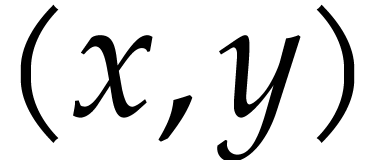painting along its way. As every other turtle of its kind, ours has a pen it uses to paint the canvas. The turtle has its pen down by default but we will see later how to change this. Finally, home is just a handy shortcut to be able to tell the turtle to (go home).

 Scheme] (define (go where) (let* ((xfinal (car where)) (yfinal (cadr where)) (ret ‘(line ,(pos) ,(point xfinal yfinal)))) (set! _posx xfinal) (set! _posy yfinal) ret))

 Scheme] (define home '(0 0))

Our turtle must turn too. We can tell it to with (turn angle), where angle is measured in degrees and taken in positive direction: a positive angle rotates to the left –counterclockwise– and a negative one to the right –clockwise–. Nevertheless, the turtle prefers the simpler instructions (right angle), (left angle). You can also force the turtle to point in a given direction with (look angle) and use any of north, south, east, west.

 Scheme] (define (turn a) (if (number? a) (with b (modulo (+ _ang a) 360) (set! _ang b))) '())

 Scheme] (define (right a) (turn (* -1 a)))

 Scheme] (define (left a) (turn a))

 Scheme] (define (look where) (if (number? where) (set! _ang (modulo where 360))) '())

 Scheme] (define north 90)

 Scheme] (define south 270)

 Scheme] (define east 0)

 Scheme] (define west 180)

You might have noticed that we explictly return the empty list after some procedures. This is because we will later be sending the output of all our drawing and positioning routines to a procedure called to-canvas used to write to the canvas. This follows the following:

Contract. Every drawing function must return something. If the procedure to-canvas receives no argument the contents of the canvas will be deleted. We agree to return a list with new content to be added or the empty list '() if nothing must be drawn

The commands (forward distance), (backward distance) move the turtle (forwards or backwards) this distance in the direction it is currently looking. These functions use go, and to compute the final coordinates we use custom trigonometric functions _sin, _cos using rationalize, that finds the nearest rational number differing less than a given constant eps. Compare (_sin pi) with the horrible (sin pi) and you'll understand. However, the difference between the drawings using the normal trigonometric functions and the custom ones is negligible. In the default scale the diameter of a pixel is less thanunits and the deviation after some movement is of the order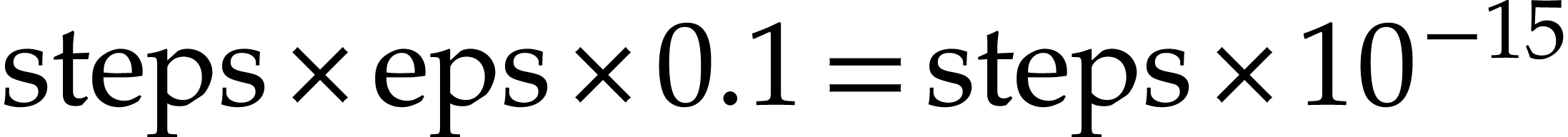. That is, the turtle would have to walk more than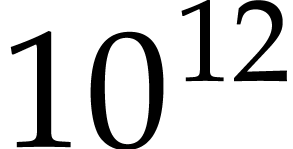units before we could notice any difference.

 Scheme] (define pi 3.14159265358979323846264338328)

 Scheme] (define eps 1e-15)

 Scheme] (define (_sin rad) (rationalize (sin rad) eps))

 Scheme] (define (_cos rad) (rationalize (cos rad) eps))

 Scheme] (define (forward dist) (let* ((rad (/ (* _ang pi) 180)) (xdist (+ _posx (* dist (_cos rad)))) (ydist (+ _posy (* dist (_sin rad))))) (go (list xdist ydist))))

 Scheme] (define (backward dist) (forward (* -1 dist)))

Let's now write some extra routines for drawing figures. We start with a rectangle using the TeXmacs graphics primitive line, then in circle we compute three suitable points to draw a circle centered at the current position of the turtle and radius r, using the primitive carc, which draws the circle defined by three points.

 Scheme] (define (rectangle n m) (let ((p1 (pos)) (p2 (point (+ _posx n) _posy)) (p3 (point (+ _posx n) (+ _posy m))) (p4 (point _posx (+ _posy m)))) ‘(line ,p1 ,p2 ,p3 ,p4 ,p1)))

 Scheme] (define (circle r) (let ((p1 (point (+ _posx r) _posy)) (p2 (point _posx (+ _posy r))) (p3 (point (- _posx r) _posy))) ‘(carc ,p1 ,p2 ,p3)))

Let's try these out! First we need to build a TeXmacs tree out of our lists. This we do with the routine (plot draw), which takes the output of a drawing routine as its argument to draw. If you don't see the results, remember that you have to activate Focus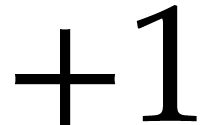Output optionsPretty tree output while inside the Scheme session.

 Scheme] (define (plot l) (stree->tree l))

 Scheme] (plot (rectangle 1 2))

 Scheme] (plot (circle 1))

This is already looking good, but we need some color! We will now define fill to set a background color, colorize for a foreground color. But first there are some technicalities to be dealt with: because of the way attributes are set for graphical primitives, using with tags, we have to take care of the following issue: What happens when we nest attribute assignments? This is solved with merge-with. Then we define decorate to set any attributes:

 Scheme] (define (merge-with l par val subs) (cond ((== (length l) 0) '()) ((== (length l) 1) (append (list par val) l)) ((== par (car l)) (if subs (set-car! (cdr l) val)) l) (else (let ((t (list (car l) (cadr l)))) (append t (merge-with (cddr l) par val subs))))))

 Scheme] (define (decorate l par val subs) (cond ((or (nlist? l) (null? l)) '()) ((list? (car l)) (append (list (decorate (car l) par val subs)) (decorate (cdr l) par val subs))) ((== (car l) 'with) (append '(with) (merge-with (cdr l) par val subs))) ((or (== (car l) 'line) (== (car l) 'carc) (== (car l) 'point)) (append '(with) (merge-with (list l) par val subs)))))

 Scheme] (define (fill fig bc) (decorate fig "fill-color" bc #f))

 Scheme] (define (force-fill fig bc) (decorate fig "fill-color" bc #t))

 Scheme] (define (colorize fig fc) (decorate fig "color" fc #f))

 Scheme] (define (force-colorize fig fc) (decorate fig "color" fc #t))

 Scheme] (define (width fig n) (if (> n 0) (decorate fig "line-width" (string-append (number->string n) "ln") #f) '()))

 Scheme] (define (force-width fig n) (if (> n 0) (decorate fig "line-width" (string-append (number->string n) "ln") #t) '()))

After some reverse-engineering of TeXmacs graphics we find how to change line styles as well. There seems to be no code number for the normal pen; as a consequence one cannot force this style.

 Scheme] (define (style fig n) (cond ((== n 0) fig) ((== n 1) (decorate fig "dash-style" "10" #f)) ((== n 2) (decorate fig "dash-style" "11100" #f)) ((== n 3) (decorate fig "dash-style" "1111010" #f)) (else '())))

 Scheme] (define (force-style n) (cond ((== n 0) fig) ((== n 1) (decorate fig "dash-style" "10" #t)) ((== n 2) (decorate fig "dash-style" "11100" #t)) ((== n 3) (decorate fig "dash-style" "1111010" #t)) (else '())))

We may finally test the colors:

 Scheme] (plot (fill (circle 2) "red"))

 Scheme] (plot (colorize (circle 2) "green"))

 Scheme] (plot (colorize (width (style (circle 4) 2) 2) "purple"))

One last routine. Use it like all the others.

 Scheme] (define (text str) ‘(with "text-at-valign" "center" "text-at-halign" "center" (text-at ,str ,(pos))))

## 3.The artist

It is time now for formal introductions. Here is our artist:

 Scheme] (define show-turtle? #t)

 Scheme] (define (turtle x y ang sz) (let ((points (map (lambda (t) (go (list x y)) (look (+ ang t)) (forward sz) (pos)) '(0 135 225 0)))) (go (list x y)) (append '(line) points)))

 Scheme] (define (show-turtle) (set! show-turtle? #t) (refresh-canvas))

 Scheme] (define (hide-turtle) (set! show-turtle? #f) (refresh-canvas))

As you can see, you may hide the turtle if it's bothering you or show it again by using hide-turtle or show-turtle. But notice those refresh-canvas: we need to have somewhere to draw and that we do in the next section.

Before we get into that we deal with a few more technicalities: first we provide a way to tell the turtle not to draw while moving. As announced this is done “moving the pen up”, which we achieve enclosing any drawing instructions within a call to up. This can be undone with a call to down. However this requires us to handle nested ups and downs. Meet simplify: this procedure takes as argument a list (of lists) l and flattens it into a simpler one (it takes some brackets away). It also uses a counter i to add all the ups and downs corresponding to an item in the list (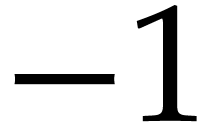for each up andfor each down). If the final sum is positive the corresponding item must not be draw and is thefore deleted from the list. The fact that we need such a function is probably a symptom of bad design: our drawing routines should have cleaner output (exercise for the reader!).

Finally we define the convenience routine draw, which calls simplify and to-canvas. draw accepts an arbitrary number of arguments, all interpreted as drawing commands.

 Scheme] (define (up l) (cons 'up (list l)))

 Scheme] (define (down l) (cons 'down (list l)))

 Scheme] (define (simplify l i) (cond ((or (null? l) (nlist? l)) '()) ((nlist? (car l)) (cond ((== (car l) 'up) (simplify (cdr l) (+ i 1))) ((== (car l) 'down) (simplify (cdr l) (- i 1))) (else (if (<= i 0) (list l) '())))) (else (append (simplify (car l) i) (simplify (cdr l) i)))))

 Scheme] (define (draw . l) (if (nnull? l) (to-canvas (simplify l 0))))

## 4.The canvas

Note. This section is merely technical and unrelated to the drawing stuff. If you are not interested in the creation of user interfaces just skip this (after executing the code!).

We finally move on to the canvas and some nice drawings. This requires some magic to open a new viewer window which involves defining a widget and a few functions. A walkthrough of this code is left for another time.

 Scheme] (define _content '())

 Scheme] (define _bgcolor "#fdfdfd")

 Scheme] (define _canvas-zoom 100) ; Percentage

 Scheme] (define (_head) (if show-turtle? ‘(colorize ,(turtle _posx _posy _ang (* 30 _zoom)) "dark green") '()))

 Scheme] (define (canvas-scale) (string-append (number->string (exact->inexact (/ _canvas-zoom 100))) "cm"))

 Scheme] (define (logo-canvas-content content head) ‘(with "bg-color" ,_bgcolor (document (with "gr-frame" (tuple "scale" ,(canvas-scale) (tuple "0.5gw" "0.5gh")) "gr-geometry" (tuple "geometry" "1par" "1par" "center") (graphics "" ,@content ,@head)))))

 Scheme] (tm-define (refresh-canvas) (refresh-now "logo-canvas"))

 Scheme] (tm-define (clear-canvas) (set! _content '()))

 Scheme] (define (set-canvas-bg col) (set! _bgcolor col) (refresh-canvas))

 Scheme] (define (set-canvas-zoom s refresh-enum?) (set! _canvas-zoom (min 400 (max 1 (string->number s)))) (refresh-canvas) (if refresh-enum? (refresh-now "logo-canvas-zoom")))

 Scheme] (tm-define (reset-canvas) (go home) (look north) (clear-canvas) (set-canvas-zoom "100" #t))

 Scheme] (define (save-canvas u) (with file (url->unix u) (if (!= (string-take-right file 3) ".ps") (set! file (string-append file ".ps"))) (with t (stree->tree (logo-canvas-content _content (_head))) (print-snippet file t))))

 Scheme] (define (toggle-turtle show?) (if show? (show-turtle) (hide-turtle)) (refresh-canvas))

 Scheme] (menu-bind canvas-background-color-menu ("Default" (set-canvas-bg "#fdfdfd")) –- (pick-background "" (set-canvas-bg answer)) –- ("Palette" (interactive-background (lambda (col) (set-canvas-bg col)) '())))

 Scheme] (tm-widget (logo-canvas-extra) // ) ; placeholder for user extension

 Scheme] (tm-widget (logo-canvas quit) (resize ("400px" "800px" "4000px") ("300px" "600px" "4000px") (vlist (refreshable "logo-canvas" (texmacs-output (stree->tree (logo-canvas-content _content (_head))) '(style "generic"))) (hlist /// (text "Background:") // (=> (balloon (icon "tm_color.xpm") "Change backround") (link canvas-background-color-menu)) /// (text "Turtle:") // (toggle (toggle-turtle answer) show-turtle?) /// // (text "Zoom (%):") // (refreshable "logo-canvas-zoom" (enum ((cut set-canvas-zoom <> #f) answer) '("10" "20" "50" "70" "80" "90" "100" "150" "200" "400") (number->string _canvas-zoom) "4em")) /// (dynamic (logo-canvas-extra)) >>> (explicit-buttons ("Save" (choose-file save-canvas "Save PostScript" "ps")) /// ("Reset" (reset-canvas)) /// ("Close" (quit)))))))

 Scheme] (tm-define (to-canvas l) ; Remember the drawing contract: ; Drawing functions such as turn that do not change _content return '() (cond ((nlist? l) (set! _content '())) ((== l '())) ((list? (car l)) (set! _content (append _content l))) (else (set! _content (append _content (list l))))) (refresh-now "logo-canvas"))

 Scheme] (tm-define (new-canvas . s) (if (nnull? s) (set! s (car s)) (set! s "Turtle's playground")) (set! _content '()) (dialogue-window logo-canvas noop s))

The way one uses this is: first create a canvas with new-canvas, then paint any drawing lists using to-canvas. In order to return to the original setup, we use (go home) then (look north) and finally (clear-canvas). A bit cumbersome, so we implemented a button for that.

We can finally test everything using new-canvas and sending the output of any of our drawing routines to the procedure to-canvas:

 Scheme] (new-canvas)

 Scheme] (to-canvas (fill (circle 2) "blue"))

## 5.Examples

We finally have all the tools to start crating some nice drawings. The next function uses map to create a colorful petal. Since on each step we want to draw two figures (a circle and a line), we need to enclose them in a list (othewise only the list corresponding to last one would be returned). In this case we must apply simplify to the final result before we can send it to the canvas, but this is already handled by draw.

 Scheme] (define (petal) (map (lambda (x y) (right x) (list (colorize (forward 10) (string-append "dark " y)) (colorize (circle 1) y))) '(20 40 60 80 60 40) '("red" "green" "blue" "yellow" "orange" "magenta")))

 Scheme] (draw (petal))

 Scheme]

With the next procedure (reps fun count) we can iterate a function fun a number count of times. Using reps with petal we have a nice flower-sort-of-thing.

 Scheme] (define (reps fun count) (if (and (number? count) (> count 0)) (append (fun) (reps fun (- count 1))) '()))

 Scheme] (draw (reps petal 6))

Colors and iterations can turn every flip into someting really nice…

 Scheme] (define (flip sz col) (map (lambda (a b) (right a) (colorize (forward (* sz (sin (/ (* b pi) 180)))) col)) '(-30 90 -120 -90) '(60 30 30 60)))

 Scheme] (define (biflip col1 col2 sz) (append (flip sz col1) (flip sz col2)))

 Scheme] (define (flower col1 col2 sz) (reps (lambda () (biflip col1 col2 sz)) 12))

 Scheme] (define (mosaic sz) (map (lambda (col1 col2 sz) (right 72) (forward sz) (flower col1 col2 sz)) '("blue" "red" "green" "magenta" "yellow") '("dark blue" "dark red" "dark green" "dark magenta" "dark yellow") '(10 10 10 10 10)))

 Scheme] (draw (mosaic 10))

 Scheme]

Another time we'll draw some classics with our turtle.

## 6.To do

These are left as exercise for the reader:

• Replace turtle with ugly gnu with bulging eyes!

• Compute the bounding box of any list to be able to stack/align arbitrary drawings.

• Simplify the interface and maybe properly document it?

• Create multiple canvases. Problem: draw receives an already evaluated list, but this list depends on the canvas on which we want to draw… so we need to delay the execution of the functions. Macros?

• Improve the drawing window, add options, maybe add a gallery…

This webpage is part of GNU TeXmacs and the larger GNU project. Verbatim copying and distribution of it is permitted in any medium, provided this notice is preserved. For more information or questions, please contact Joris van der Hoeven.

Free Software Foundation, Inc., 51 Franklin Street, Fifth Floor, Boston, MA 02111, USA